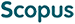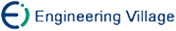姚宏亮 张一鸣 李俊照 王 浩. 动态贝叶斯网络的灵敏性分析研究[J]. 计算机研究与发展, 2014, 51(3): 536-547.
 引用本文: 姚宏亮 张一鸣 李俊照 王 浩. 动态贝叶斯网络的灵敏性分析研究[J]. 计算机研究与发展, 2014, 51(3): 536-547.Yao Hongliang, Zhang Yiming, Li Junzhao, and Wang Hao. Research on Sensitivity Analysis for Dynamic Bayesian Networks[J]. Journal of Computer Research and Development, 2014, 51(3): 536-547.
 Citation: Yao Hongliang, Zhang Yiming, Li Junzhao, and Wang Hao. Research on Sensitivity Analysis for Dynamic Bayesian Networks[J]. Journal of Computer Research and Development, 2014, 51(3): 536-547.## Research on Sensitivity Analysis for Dynamic Bayesian Networks

• 摘要: 灵敏性分析是研究复杂系统特性的一种重要方法.现有动态灵敏性分析方法都是针对特定类型的动态贝叶斯网络且计算复杂度高.为了对一般动态贝叶斯网络的灵敏性进行有效分析，提出了一种基于联合树的动态灵敏性分析算法(DSA_JT)，DSA_JT算法构建动态网络的联合树，通过消息传播建立参数与目标结点的条件概率分布在时间上的函数关系；DSA_JT将联合概率分布分解成局部概率因式形式，通过降低计算幂次提升计算效率，但计算复杂度仍然偏高.为了更有效地提高动态贝叶斯网络灵敏性分析的计算性能，在DSA_JT算法的框架上提出了DSA_BK算法，DSA_BK算法在灵敏性函数计算过程中，用子系统的概率乘积近似整个系统的联合概率，通过对接口结点局部性的边缘化操作更新模型的联合概率分布，进一步降低了计算幂次，并论证了DSA_BK算法误差的有界性.进而，通过对这两种算法过程的抽象，分别给出了动态灵敏度函数计算公式的证明，表明2种算法可以有效处理一般动态贝叶斯网络的灵敏性分析问题.最后，在上证股票网络上的实验结果显示这2种算法的有效性.

Abstract: Sensitivity analysis is an important method for researching the characteristic of complex system. The present algorithms of dynamic sensitivity analysis are used to deal with some special kinds of dynamic Bayesian network and their computation complexity is very high. Therefore, in order to handle the sensitivity analysis on general dynamic Bayesian network effectively, a new algorithm is presented based on junction-tree algorithm (DSA_JT). The function relations between parameters and conditions probability distribution of object node are established by message-propagation on junction-tree. DSA_JT can lower exponential time and reduce calculation significantly. But its computation complexity is still high. In order to further improve the computational performance, DSA_BK is introduced based on DSA_JT algorithm. DSA_BK introduces the factor idea with the product of the probability of the subsystems to approximate the probability of the whole system. DSA_BK reduces the size of the root by the local marginalisation of interface and updating the joint probability distribution of model. Compared with DSA_JT, DSA_BK can lower the computational exponential times further and significantly improves the calculation efficiency, and the error is bound to be proved. Then, based on abstracting the process of the two algorithms, the computation formulas of dynamic sensitivity function are proved, and it is shown that the two algorithms can effectively deal with sensitivity analysis of general dynamic Bayesian network. Finally, the effectiveness is proved in the experiment on the network of the Shanghai Stock./下载:  全尺寸图片 幻灯片
• 分享
• 用微信扫码二维码

分享至好友和朋友圈